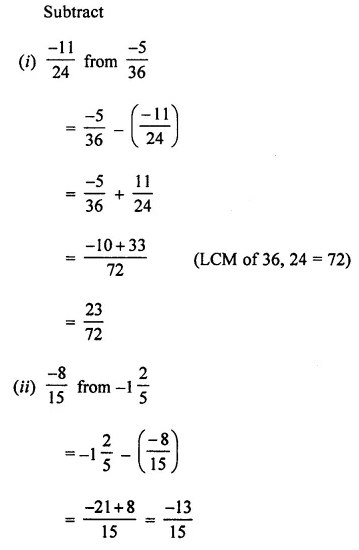ML Aggarwal Class 7 Solutions Chapter 3 Rational Numbers Check Your Progress for ICSE Understanding Mathematics acts as the best resource during your learning and helps you score well in your exams.

## ML Aggarwal Class 7 Solutions for ICSE Maths Chapter 3 Rational Numbers Check Your Progress

Question 1.
Write five rational numbers equivalent to $$\frac { 5 }{ -11 }$$
Solution:Question 2.
Express $$\frac { 9 }{ -15 }$$ as a rational number with:
(i) denominator 5
(ii) numerator -12
(iii) denominator 30
Solution: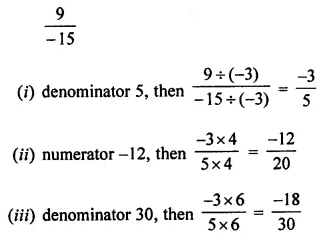Question 3.
Write each of the following numbers in standard form: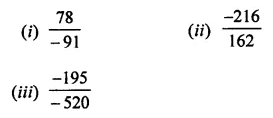Solution: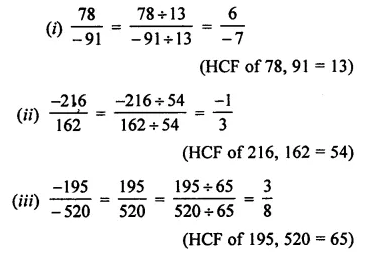Question 4.
Which of the following are pairs of equivalent rational numbers?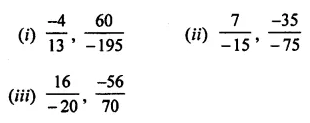Solution: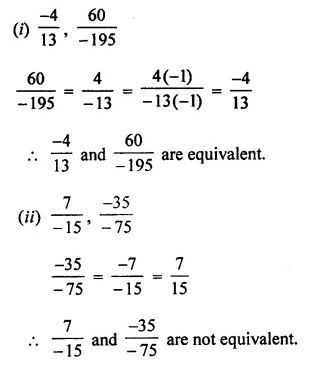Question 5.
Arrange the following rational numbers in ascending order:Solution: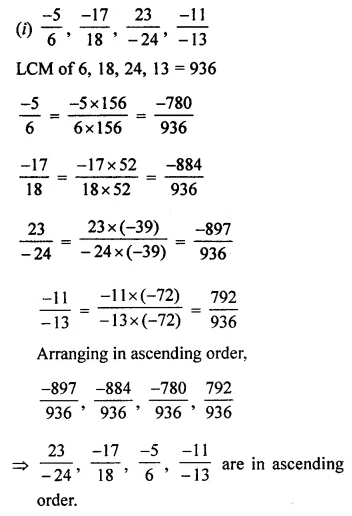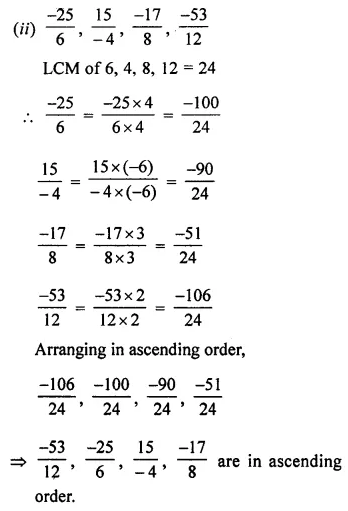Question 6.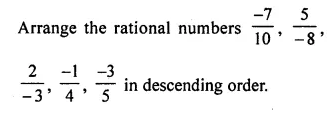Solution:Question 7.
Insert five rational numbers between $$\frac { -3 }{ 5 }$$ and $$\frac { -1 }{ 2 }$$.
Solution: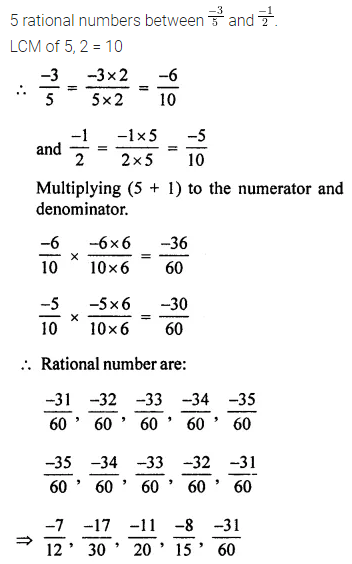Question 8.
Find the sum: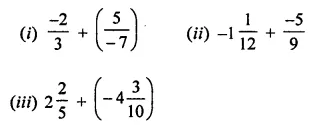Solution:Question 9.
Subtract: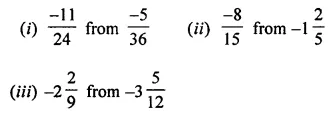Solution: